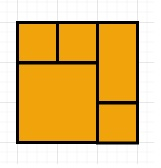# Perfect Rectangle in C++

Suppose we have N axis-aligned rectangles, we have to check whether they all together form an exact cover of a rectangular region or not. Here each rectangle is represented as a bottom-left point and a top-right point. So a unit square is represented as [1,1,2,2]. (bottom-left point is (1, 1) and top-right point is (2, 2)).

So, if the input is like rectangles = [[1,1,3,3],[3,1,4,2],[3,2,4,4],[1,3,2,4],[2,3,3,4]], then the output will be true as all 5 rectangles together form an exact cover of a rectangular region.To solve this, we will follow these steps −

• Define one set visited

• area := 0

• x2 := -inf, x1 := inf

• y2 := -inf, y1 := inf

• for each r in given list re −

• x1 := minimum of r and x1

• x2 := maximum of r and x2

• y1 := minimum of r and y1

• y2 := maximum of r and y2

• area := area + ((r - r) * (r - r))

• s1 := r concatenate r

• s2 := r concatenate r

• s3 := r concatenate r

• s4 := r concatenate r

• if s1 is visited, then −

• delete s1 from visited

• Otherwise

• insert s1 into visited

• if s2 is visited, then −

• delete s2 from visited

• Otherwise

• insert s2 into visited

• if s3 is visited, then −

• delete s3 from visited

• Otherwise

• insert s3 into visited

• if s4 is visited, then −

• delete s4 from visited

• Otherwise

• insert s4 into visited

• s1 := concatenate x1 and y1

• s2 := concatenate x2 and y1

• s3 := concatenate x1 and y2

• s4 := concatenate x2 and y2

• if s1, s2, s3, s4 all are not visited, then

• return false

• return true when area is same as ((x2 - x1) * (y2 - y1))

## Example

Let us see the following implementation to get better understanding −

Live Demo

#include <bits/stdc++.h>
using namespace std;
class Solution {
public:
bool isRectangleCover(vector<vector<int>> &re) {
unordered_set<string> visited;
int area = 0;
int x2 = INT_MIN;
int x1 = INT_MAX;
int y2 = INT_MIN;
int y1 = INT_MAX;
for (auto &r : re) {
x1 = min(r, x1);
x2 = max(r, x2);
y1 = min(r, y1);
y2 = max(r, y2);
area += (r - r) * (r - r);
string s1 = to_string(r) + to_string(r);
string s2 = to_string(r) + to_string(r);
string s3 = to_string(r) + to_string(r);
string s4 = to_string(r) + to_string(r);
if (visited.count(s1)) {
visited.erase(s1);
}
else
visited.insert(s1);
if (visited.count(s2)) {
visited.erase(s2);
}
else
visited.insert(s2);
if (visited.count(s3)) {
visited.erase(s3);
}
else
visited.insert(s3);
if (visited.count(s4)) {
visited.erase(s4);
}
else
visited.insert(s4);
}
string s1 = to_string(x1) + to_string(y1);
string s2 = to_string(x2) + to_string(y1);
string s3 = to_string(x1) + to_string(y2);
string s4 = to_string(x2) + to_string(y2);
if (!visited.count(s1) || !visited.count(s2) || !visited.count(s3) || !visited.count(s4) || visited.size() != 4)
return false;
return area == (x2 - x1) * (y2 - y1);
}
};
main() {
Solution ob;
vector<vector<int>> v = {{1, 1, 3, 3}, {3, 1, 4, 2}, {3, 2, 4, 4}, {1, 3, 2, 4}, {2, 3, 3, 4}};
cout << (ob.isRectangleCover(v));
}

## Input

{{1, 1, 3, 3}, {3, 1, 4, 2}, {3, 2, 4, 4}, {1, 3, 2, 4}, {2, 3, 3, 4}}

## Output

1